# Assess Power-Supply Noise Rejection in Low-Jitter PLL Clock Generators

### Abstract

This article discusses the effects of power-supply noise interference on PLL-based clock generators, and describes several measurement techniques for evaluating the resulting deterministic jitter (DJ). Derived relationships show how frequency-domain spur measurements can be used to evaluate timing-jitter behavior. Laboratory bench-test results are used to compare the measurement techniques, and demonstrate how to reliably assess the power-supply noise rejection (PSNR) performance of a reference clock generator.

This article was also featured in Maxim's Engineering Journal, vol. 66 (PDF, 3.07MB).

A version of this article was published online by Electronic Design magazine, March 27, 2009.

Clock generators that employ PLLs are widely used in network equipment for generating high-precision and low-jitter reference clocks or for maintaining a synchronized network operation. Most clock oscillators give their jitter or phase-noise specification using an ideal, clean power supply. In a practical system environment, however, the power supply can suffer from interference due to on-board switching supplies or noisy digital ASICs. To achieve the best performance in a system design, it is important to understand the effects of such interference.

First we will examine the basic power-supply noise rejection (PSNR) characteristics of a PLL-based clock generator. We will then explain how to extract timing-jitter information from measurements taken in the frequency domain. These techniques are then applied and several different measurement methodologies are compared using lab bench testing. Finally, we will summarize the merits of the preferred approach.

### PSNR Characteristics of PLL Clock Generators

A typical PLL clock generator is shown in Figure 1. Since the output driver can have very different PSNR performance for different types of logic interfaces, the following analysis will focus on the impact of supply noise on the PLL itself.Figure 1. A typical topology for a PLL clock generator.

Figure 2 shows the PLL phase model. The model assumes that the power supply noise, VN, is injected into the PLL/VCO, and that the divide ratios, M and N, are set to 1.Figure 2. The phase model for a PLL.

The PLL closed-loop transfer function from VN(s) to ΦO(s) is given by:For a typical 2nd-order PLL: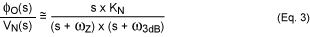Here ω3dB is the PLL 3dB bandwidth, ωZ is the PLL zero frequency, and ωZ << ω3dB.

Equation 3 demonstrates that in a PLL clock generator the power-supply noise is rejected by 20dB/dec, when the power-supply interference (PSI) frequency is greater than the PLL 3dB bandwidth. For PSI frequencies between ωZ and ω3dB, the output clock phase varies with the PSI amplitude as:As an example, Figure 3 shows the PSNR characteristics of a PLL for two different settings of the PLL's 3dB bandwidth.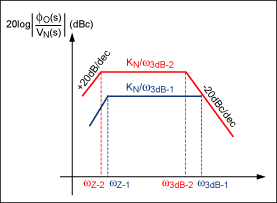Figure 3. Typical PLL PSNR characteristics.

### Conversion of Power Spectrum Spurs to DJ

When a single-tone sinusoidal signal, fM, is applied to the power supply of a PLL, it produces a narrow-band phase modulation at the clock output. This phase modulation can be generally described using Fourier series representation:Here β is the modulation index representing the maximum phase deviation. For a small index modulation (β << 1), the Bessel function can be approximated as: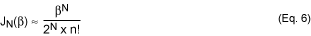Here n = 0 represents the carrier itself. When n = ±1, the phase-modulated signal is given by: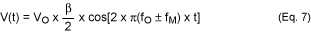When measuring the double-sideband power spectrum SV(f), if variable x represents the level difference between the carrier at fO and the fundamental sideband tone at fM, then:Since β is the maximum phase deviation in radians, the peak-to-peak DJ caused by this small index phase modulation can be derived as:The above analysis assumes that there is no amplitude modulation contributing to the tone at fM. In reality, both amplitude and phase modulation can be generated, thus reducing the accuracy of this approach.

### Conversion of Phase-Noise Spectrum Spurs to DJ

There is a way to avoid the amplitude modulation effect when measuring the power spectrum SV(f). One can instead calculate the DJ by measuring the spur in the phase-noise spectrum, while applying a single-tone sinusoidal interference on the supply. With the variable y (dBc) representing the measured single-sideband-phase spurious power at frequency offset fM, the resultant phase deviation ΔΦ(radRMS) can be derived as: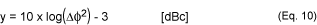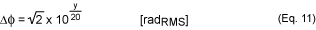It should be noted that the single-sideband-phase spectrum in the above analysis is not the folded version of the double-sideband spectrum. That is the reason for the 3dB component in Equation 10. Figure 4 shows the relationship between the DJ and the phase spurious power given by Equation 12.Figure 4. DJ vs. phase spurious power.

### PSNR Measurement Techniques

This next section demonstrates five different ways of measuring the PSNR of a clock source. The MAX3624 low-jitter clock generator serves as an example. The measurement setup shown in Figure 5 uses a function generator to inject a sinusoidal signal onto the power supply of the MAX3624 evaluation (EV) board. The amplitude of the single-tone interference is measured directly at the VCC pin close to the IC. A limiting amplifier, the MAX3272, is used to remove amplitude modulation; it is followed by a balun that converts the differential output into a single-ended signal for driving the different test equipment. To compare the results from different tests, all the measurements were done under the following conditions:

1. Clock output frequency: fO = 125MHz
2. Sinusoidal modulation frequency: fM = 100kHz
3. Sinusoidal signal amplitude: 80mVP-PFigure 5. PSNR measurement setup.

### Method 1. Power Spectrum Measurement

When observed on a power spectrum analyzer, the narrow-band phase modulation appears as two sidebands around the carrier. Figure 6 shows this case when viewed using the spectrum monitor function of the Agilent® E5052. The measured first sideband amplitude relative to carrier amplitude is -53.1dBc, which translates to 11.2psP-P DJ, according to Equation 9.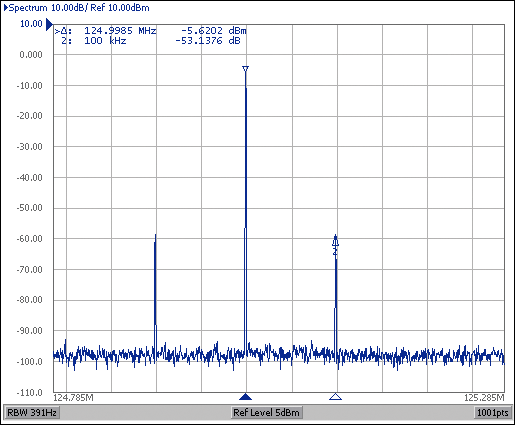Figure 6. Measured power spectrum.

### Method 2. SSB Phase Spurious Measurement

On a phase-noise analyzer, the PSI will manifest itself as a phase spur relative to the carrier. The measured phase-noise spectrum is plotted in Figure 7. The phase spurious power at 100kHz is -53.9dBc, which translates to 10.2psP-P DJ using Equation 12.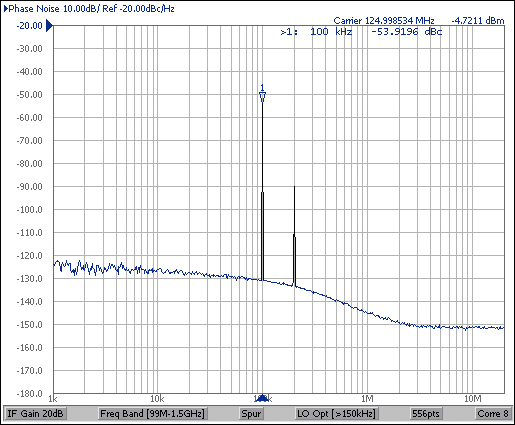Figure 7. Measured SSB phase noise and spur.

### Method 3. Phase Demodulation Measurement

Using the Agilent E5052 signal analyzer, the phase-demodulated sinusoidal signal at 100kHz is measured directly as shown in Figure 8, which gives the maximum phase deviation from its ideal position. The peak-to-peak phase deviation is 0.47°, which translates to 10.5psP-P at an output frequency of 125MHz.Figure 8. MAX3624 phase demodulation signal.

### Method 4. Real-Time Scope Measurement

In a time domain measurement, the DJ caused by PSI can be obtained by measuring the time interval error (TIE) histogram. On a real-time scope, the clock-output TIE distribution will appear as a sinusoidal probability density function (PDF) when a single-tone interference is injected into the PLL. The DJ can be estimated using the dual-Dirac model¹ by measuring the peak distance between the mean of two Gaussian distributions from the TIE histogram. Figure 9 shows the measured TIE histogram using the Agilent Infiniium DSO81304A 40GSa/s real-time scope. The measured peak separation is 9.4ps.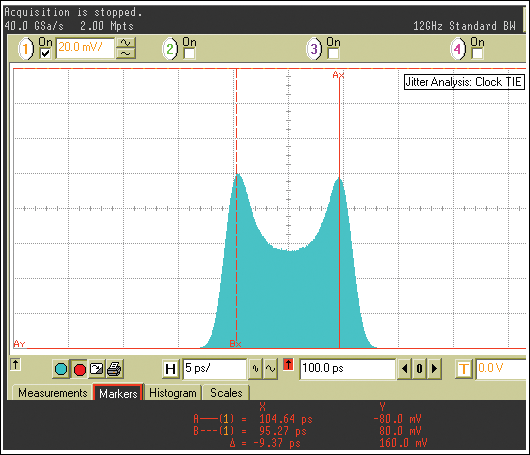Figure 9. Measured TIE histogram.

It should be noted that the memory depth of the real-time scope may limit the low sinusoidal modulation frequency that can be applied to the PLL supply. For example, if the test equipment has a memory depth of 2Msps when the sample rate is set to 40Gsps, it would only be able to capture jitter frequency components down to 20kHz.

### Method 5. Sampling Scope Measurement

When a sampling scope is used, a synchronous trigger signal is required for analyzing the clock jitter under test. Two triggering methods can be used for TIE measurements.

The first method is to apply a low-jitter reference clock to the input of the PLL clock generator; use the same clock source as the trigger for the sampling scope. Figure 10a shows the measured TIE histogram, which gives a peak spacing of 9.2ps. The advantage of triggering with a reference clock is that the measured TIE histogram peak separation is independent of the horizontal time delay from the trigger position. However, the measured TIE histogram can be affected by the triggering clock jitter. Therefore, it is important to use a clock source that has much lower jitter than the clock generator device under test.

The alternate approach uses self-triggering to eliminate the impact of triggering clock jitter. In this case, the output of the clock generator under test is separated into two identical signals using a power splitter. One signal is applied to the data input of the sampling scope, another signal to the trigger input. Since the triggering signal contains the same DJ as the test signal, the histogram peak separation varies when the horizontal position of the scope's main time base is swept through one period of the sinusoidal modulation frequency. At a horizontal position of one-half period of the modulation signal, the peak separation on the TIE histogram will be twice the DJ from the test signal. Figure 10b shows the measured MAX3624 TIE histogram when the horizontal time delay is set to 5µs. The estimated TIE peak separation is 19ps, which gives an equivalent DJ of 9.5psP-P.

Figure 10c shows the measured TIE histogram peak spacing at a different horizontal time delay from the trigger point. For comparison, the TIE result is also shown when the sampling scope is triggered by a reference clock input.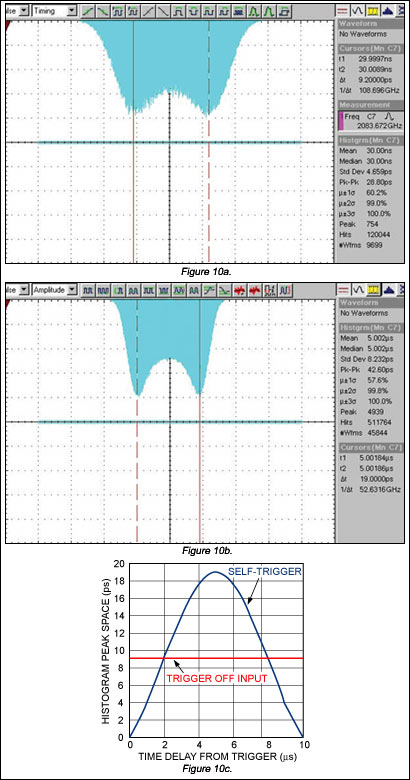More detailed image
(PDF, 69kB)
Figure 10. TIE histograms are shown for various trigger conditions: triggered by REF_IN (a); self-triggered, td = 5µs (b); and peak spacing vs. time delay from trigger (c).

### Measurement Summary

Table 1 summarizes the measured DJ at the MAX3624 125MHz clock output. Data was gathered using the different methods discussed above. It should be noted that the DJ measured using a dual-Dirac approximation from the TIE histogram is slightly smaller than the DJ obtained from the frequency-domain spectral analysis. This difference is caused by the process of convolution of the sinusoidal jitter (SJ) PDF with Gaussian distribution of the random jitter component.¹ Therefore, the DJ extracted from the dual-Dirac model is only an estimation; it should only be applied when the standard deviation of the random jitter is much smaller than the distance between the two peak separations of the jitter histogram.

Table 1. DJ Comparison*

 Measurement Methods DJ (psP-P) Power Spectrum 11.2 SSB Phase Spurious 10.3 Phase Decomposition 10.5 Real-Time Scope 9.4 Sampling Scope(Reference Triggered) 9.2 Sampling Scope(Self-Triggered) 9.5
*80mVP-P, 100kHz sinusoidal signal on the supply.

### Conclusion

For the relatively large interference used in the examples, the results were well correlated. However, when the level of interference drops relative to the random jitter, the time-domain methods become less accurate. Furthermore, if the clock signal is corrupted by amplitude modulation, measurements using a power spectrum analyzer become unreliable. Therefore, of all the methods presented, the phase spur power measurement using a phase-noise analyzer is the most accurate and convenient way to characterize the PSNR of a clock generator. The same method can be extended for evaluating the DJ aspect caused by other spurious products appearing on the phase-noise spectrum.

Reference
Agilent white paper, "Jitter Analysis: the dual-Dirac Model, RJ/DJ, and Q-Scale."